# How to Calculate and Solve for Radiosity | Radiation Heat Transfer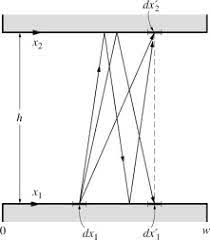To compute for radiosity, three essential parameters are needed and these parameters are Total Emissive Power (e), Reflectivity (ρ) and Total Irradiation (G).

J = e + ρG

Where:

e = Total Emissive Power
ρ = Reflectivity

Let’s solve an example;
Find the radiation heat transfer when the total emissive power is 12, the reflectivity is 10 and the total irradiation is 20.

This implies that;

e = Total Emissive Power = 12
ρ = Reflectivity = 10
G = Total Irradiation = 20

J = e + ρG
J = 12 + (10)(20)
J = 12 + 200
J = 212

Therefore, the radiosity is 212 W/m².

Calculating the Total Emissive Power when the Radiosity, the Reflectivity and the Total Irradiation is Given.

e = J – ρG

Where:

e = Total Emissive Power
ρ = Reflectivity

Let’s solve an example;
Find the total emissive power when the radiation heat transfer is 32, the reflectivity is 10 and the total irradiation is 2.

This implies that;

J = Radiation Heat Transfer = 32
ρ = Reflectivity = 10
G = Total Irradiation = 2

e = J – ρG
e = 32 – (10)(2)
e = 32 – 20
e = 12

Therefore, the total emissive power is 12.

Calculating the Reflectivity when the Radiosity, the Total Emissive Power and the Total Irradiation is Given.

ρ = J – e / G

Where:

ρ = Reflectivity
e = Total Emissive Power

Let’s solve an example;
Find the reflectivity when the radiation heat transfer is 28, the total emissive power is 10 and the total irradiation is 4.

This implies that;

J = Radiation Heat Transfer = 28
e = Total Emissive Power = 10
G = Total Irradiation = 4

ρ = J – e / G
ρ = 28 – 10 / 4
ρ = 18 / 4
ρ = 4.5

Therefore, the reflectivity is 4.5.

Calculating the Total Irradiation when the Radiosity, the Total Emissive Power and the Reflectivity is Given.

G = J – e / ρ

Where:

e = Total Emissive Power
ρ = Reflectivity

Let’s solve an example;
Find the total irradiation when the radiation heat transfer is 44, the total emissive power is 14 and the reflectivity is 2.

This implies that;

J = Radiation Heat Transfer = 44
e = Total Emissive Power = 14
ρ = Reflectivity = 2

G = J – e / ρ
G = 44 – 14 / 2
G = 30 / 2
G = 15

Therefore, the total irradiation is 15.

Nickzom Calculator – The Calculator Encyclopedia is capable of calculating the radiosity.

To get the answer and workings of the radiosity using the Nickzom Calculator – The Calculator Encyclopedia. First, you need to obtain the app.

You can get this app via any of these means:

To get access to the professional version via web, you need to register and subscribe for NGN 2,000 per annum to have utter access to all functionalities.
You can also try the demo version via https://www.nickzom.org/calculator

Apple (Paid) – https://itunes.apple.com/us/app/nickzom-calculator/id1331162702?mt=8
Once, you have obtained the calculator encyclopedia app, proceed to the Calculator Map, then click on Materials and Metallurgical under Engineering.Now, Click on Radiation Heat Transfer under Materials and Metallurgical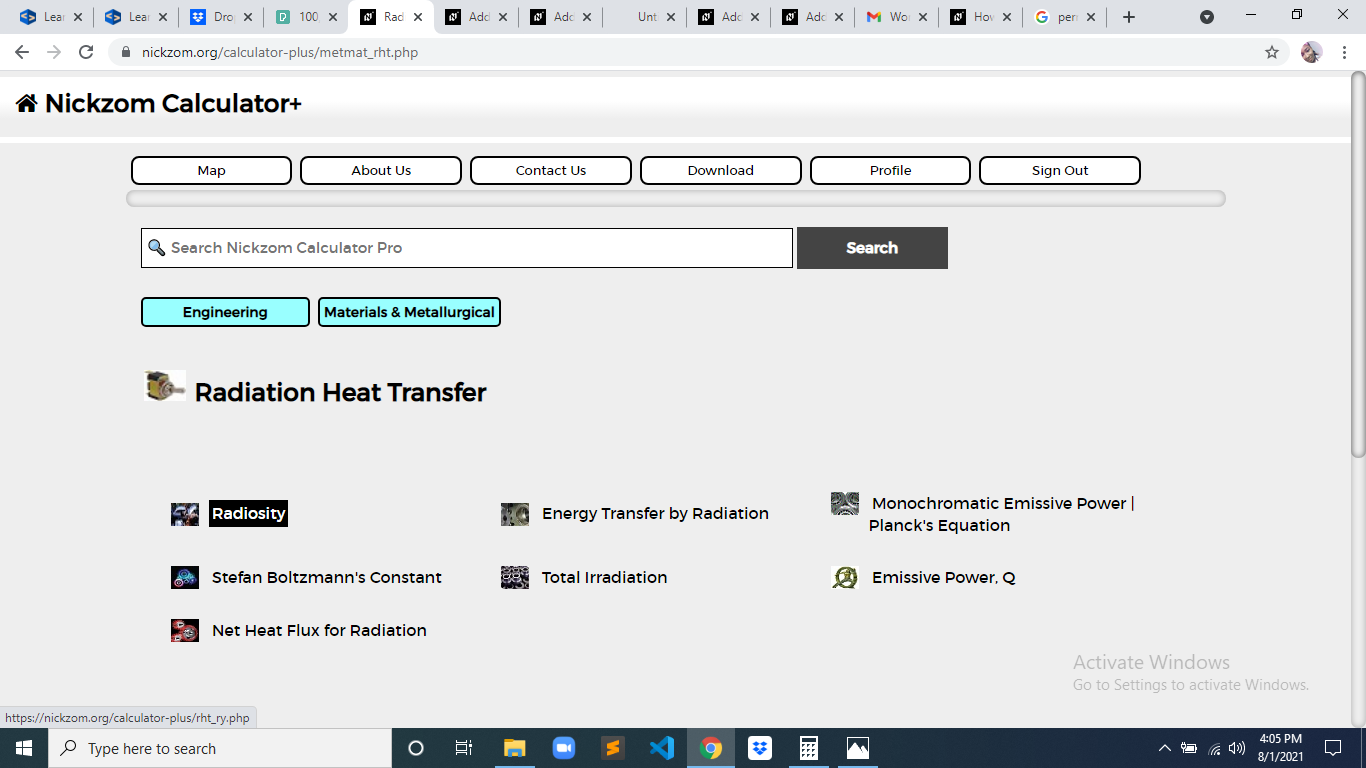The screenshot below displays the page or activity to enter your values, to get the answer for the radiosity according to the respective parameter which is the Total Emissive Power (e), Reflectivity (ρ) and Total Irradiation (G).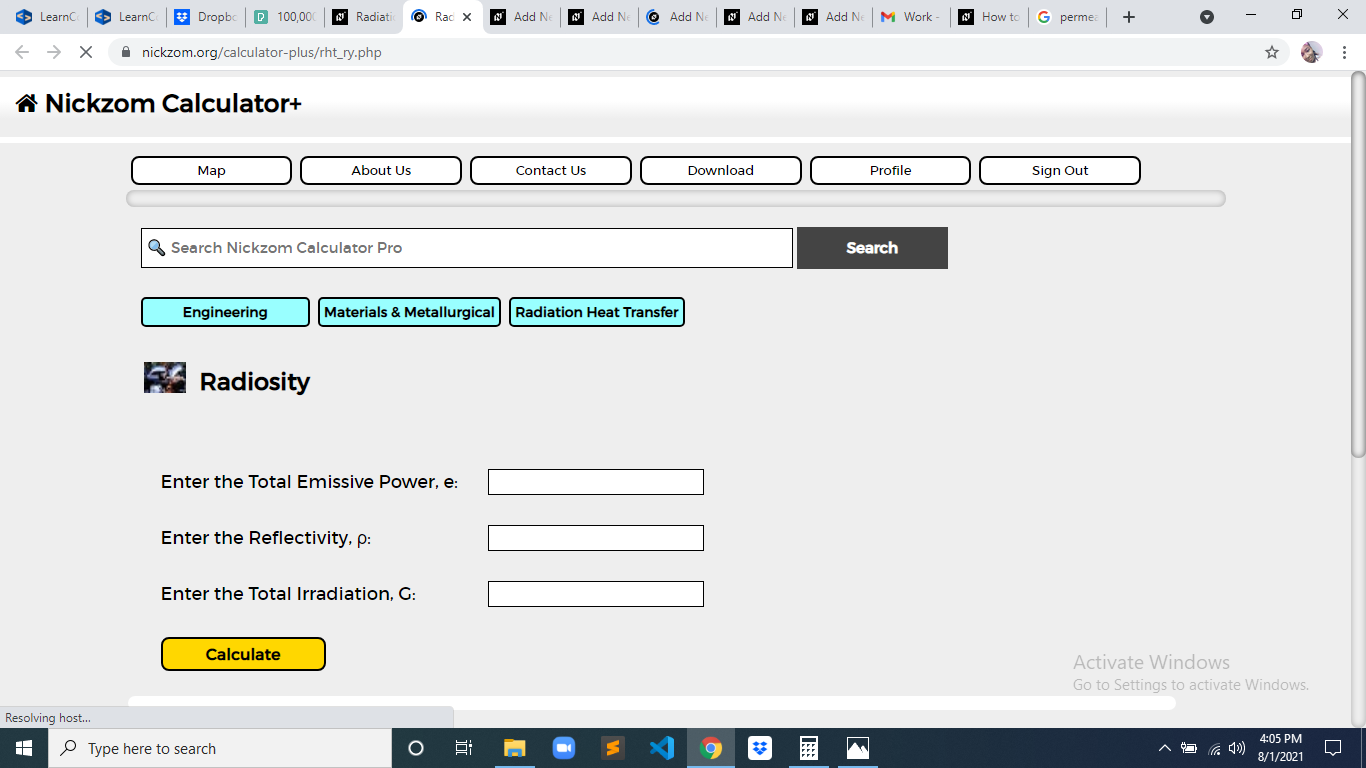Now, enter the values appropriately and accordingly for the parameters as required by the Total Emissive Power (e) is 12, Reflectivity (ρ) is 10 and Total Irradiation (G) is 20.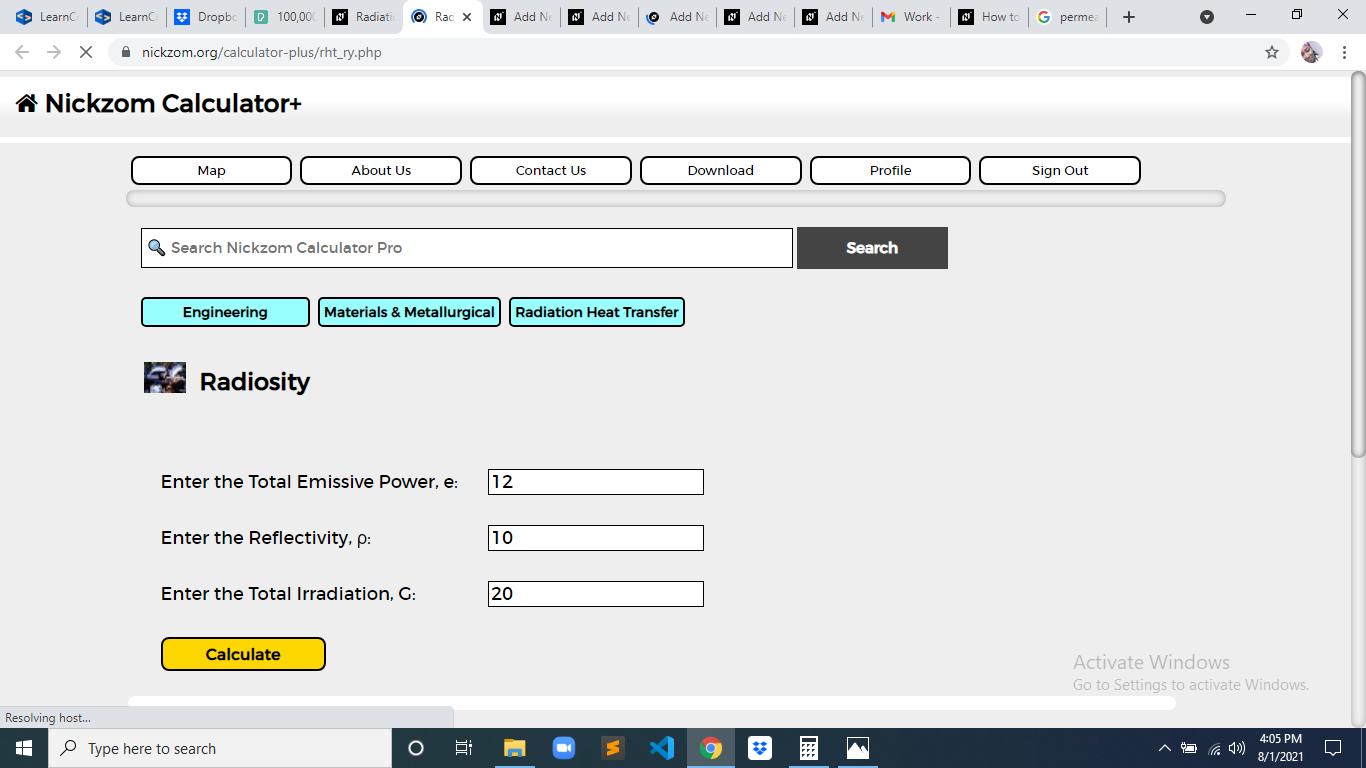Finally, Click on Calculate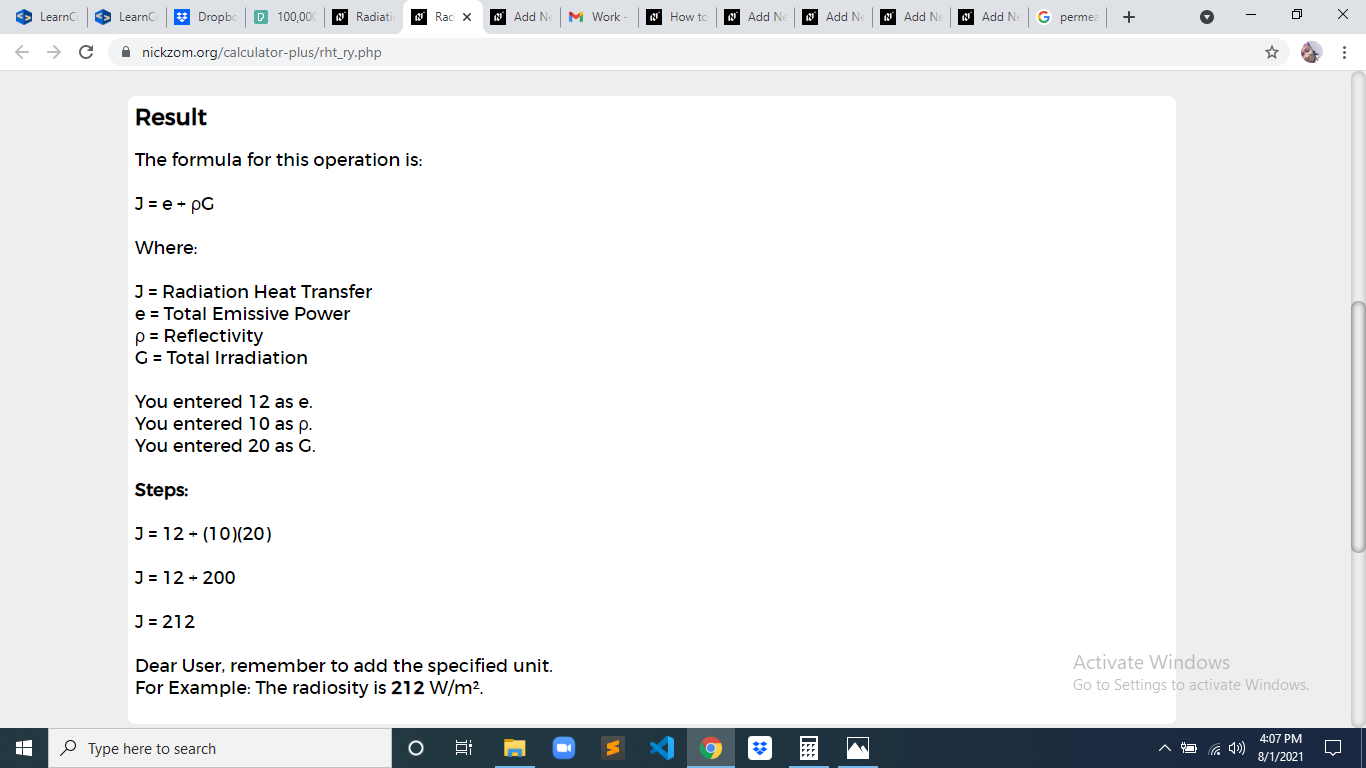As you can see from the screenshot above, Nickzom Calculator– The Calculator Encyclopedia solves for the radiosity and presents the formula, workings and steps too.